# 数学公式编辑神器-Mathpix Snipping Tool

## theme: smartblue## 编辑常见公式

1、勾股定理

$$a^2 + b^2 = c^2$$2、三角和差公式

$$sin(\alpha + \beta) = sin\alpha cos\beta + cos\alpha sin\beta$$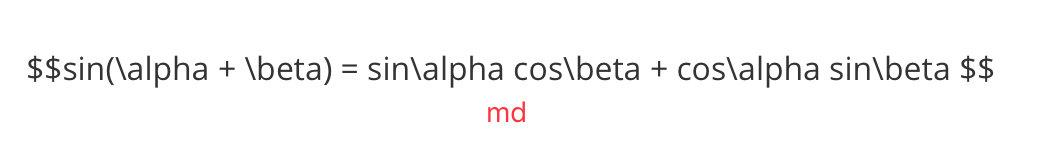$$cos(\alpha + \beta) = cos\alpha cos\beta - sin\alpha sin\beta$$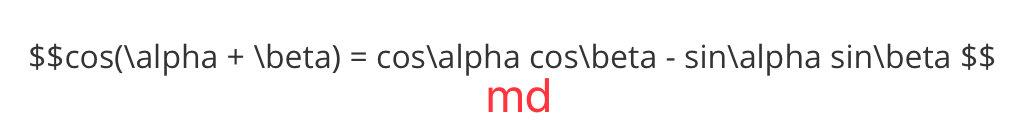3、三角函数积分公式

$$cosx = \frac {1-u^2}{1 + u^2}$$$$f(x)=f(a)+\frac{f^{\prime}(a)}{1 !}(x-a)+\frac{f^{(2)}(a)}{2 !}(x-a)^{2}+\cdots+\frac{f^{(n)}(a)}{n !}(x-a)^{n}+\frac{f^{(n+1)}(\theta)}{(n+1) !}(x-a)^{(n+1)}$$

## Mathpix Snipping Tool## 谁在用？## 支持哪些软件格式？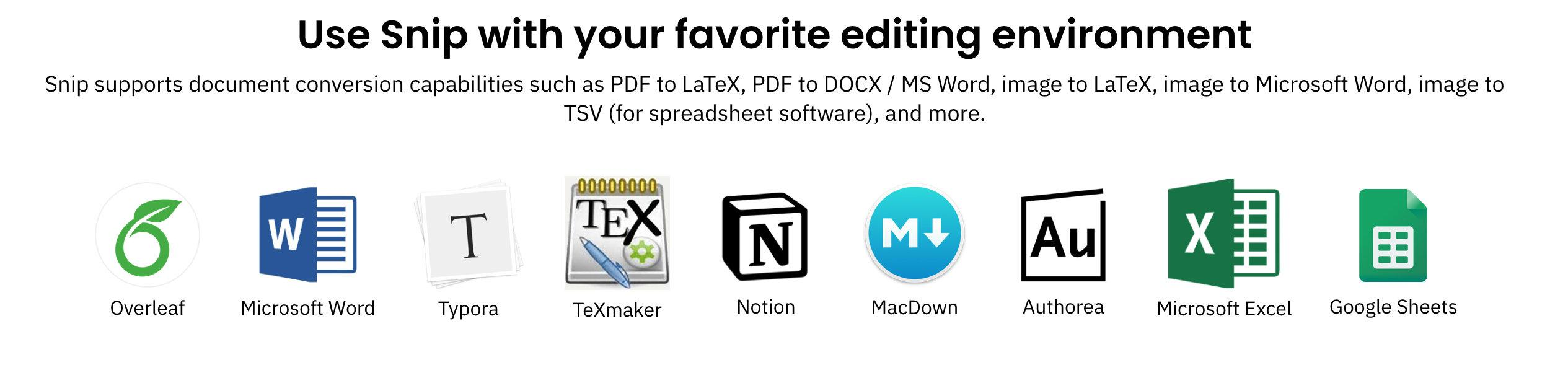## 效果如何？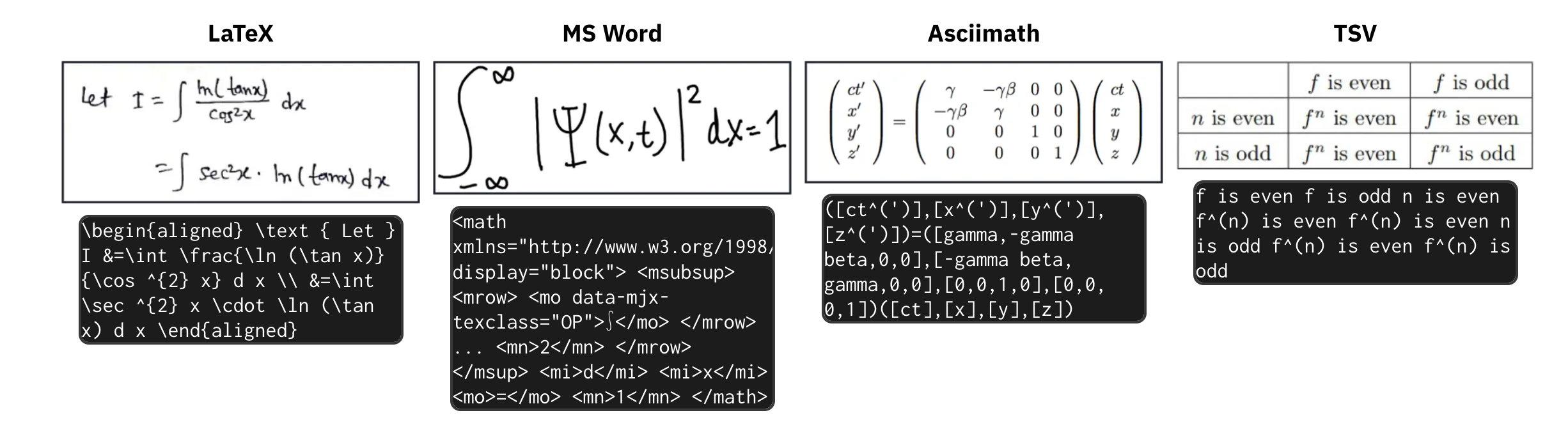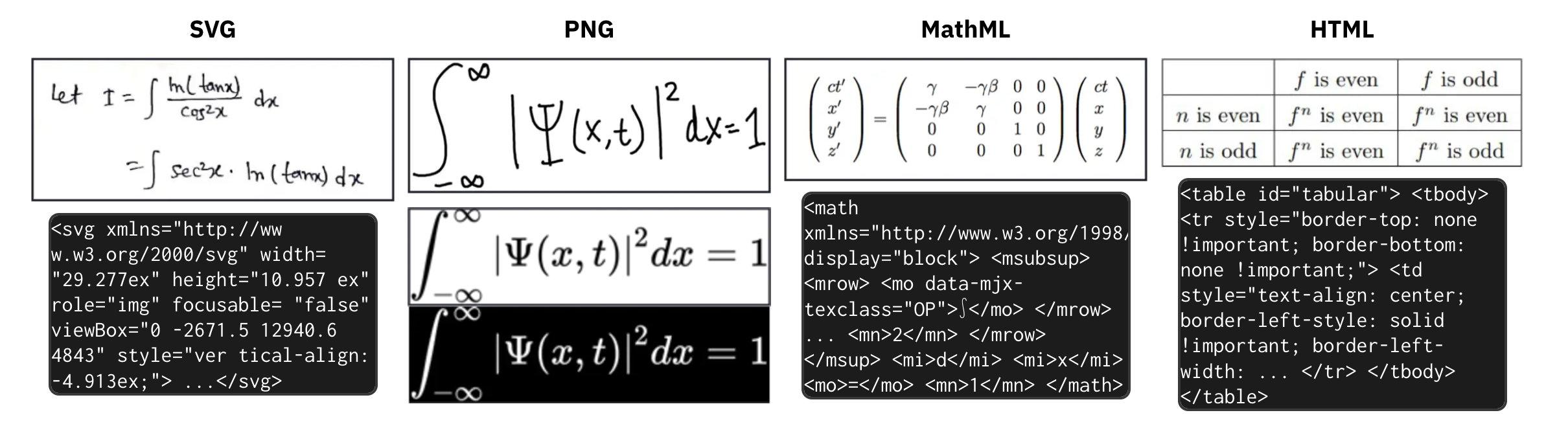## 如何使用

1、打开Mathpix Snipping Tool

2、对公式部分进行圈选截图

3、复制Markdown源码即可

1、Typora：将复制的Markdown源码放入两对$$中间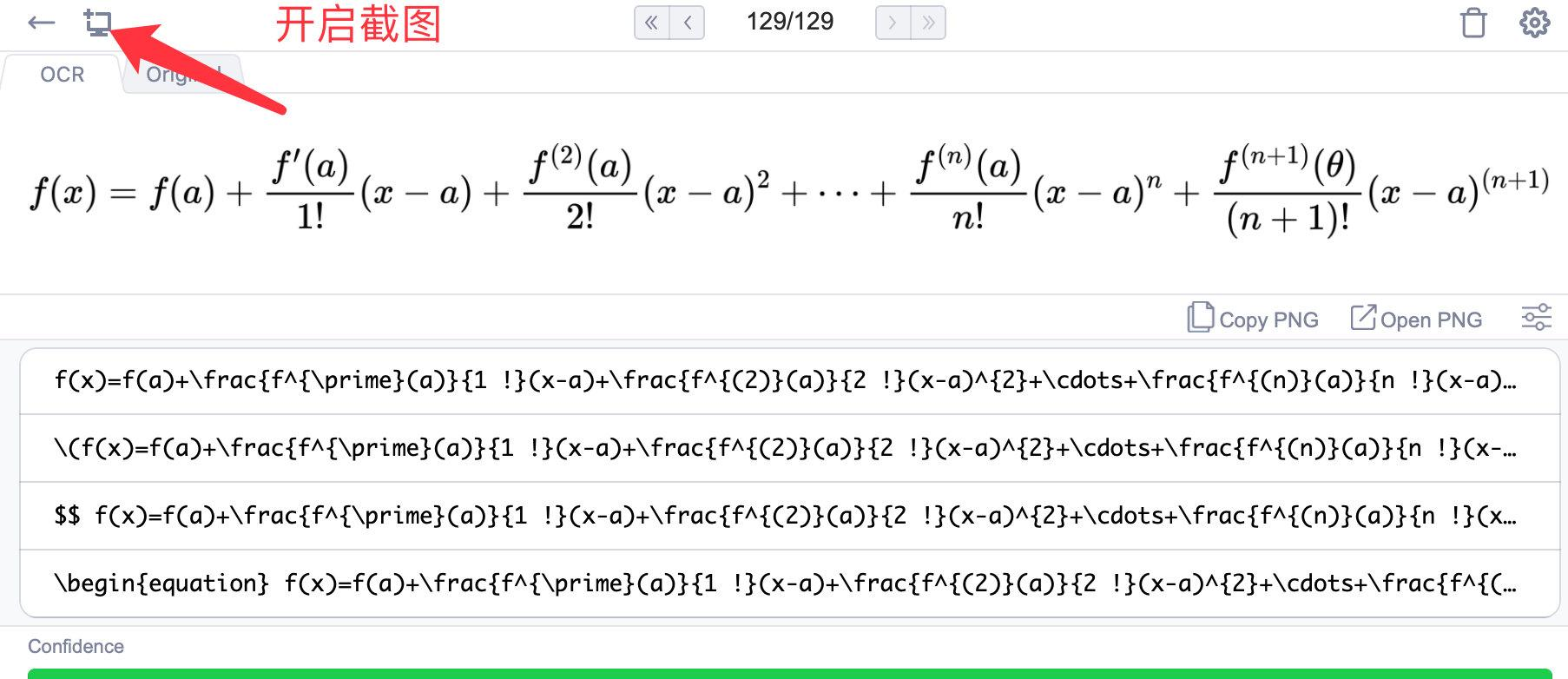$$f(x)=f(a)+\frac{f^{\prime}(a)}{1 !}(x-a)+\frac{f^{(2)}(a)}{2 !}(x-a)^{2}+\cdots+\frac{f^{(n)}(a)}{n !}(x-a)^{n}+\frac{f^{(n+1)}(\theta)}{(n+1) !}(x-a)^{(n+1)}

2、jupyter notebook中使用看视频：

「其他文章」